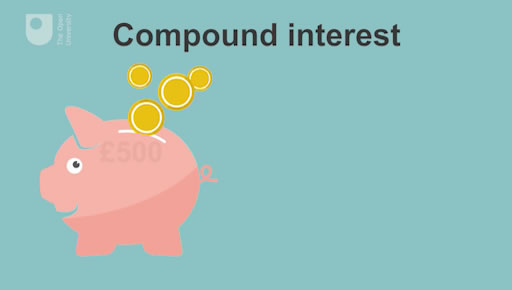Managing my money for young adults

Start this free course now. Just create an account and sign in. Enrol and complete the course for a free statement of participation or digital badge if available.

Free course

# 10 Calculating interest on savings accounts

Interest payments are computed on a per annum basis. This means that interest earnings are based on the length of time you keep money in your account. So, for example, in cash terms the interest for a 6-month period will be half that for a full year.

If you have an average balance of £1000 in your account and the interest rate is 3% per annum then you will receive an interest payment for the year of £1000 x 3/100 = £30. The balance on your account will rise to £1030.

If the balance stays at £1030 over the next year and the interest rate paid stays at 3% per annum then the interest paid will be £1030 x 3/100 = £30.90. This will mean that the balance on your account will rise to £1060.90.

The interest is higher for the second year because interest has been paid not only on the original balance of £1000 but also on the £30 interest earned in the first year. This process, which is very important to understand when saving or borrowing money, is known as ‘compounding’. Interest that is paid on interest previously paid is known as ‘compound interest’.

Some accounts pay interest monthly, some quarterly and some half-yearly. But by far the majority of accounts pay interest annually. You should check which rate applies to your account.

The interest paid to you will be based on the average balance of your account during the interest period. It is not paid on the basis of the money you have in your account at the date that the interest is computed. So if the amount of interest paid to you looks a little odd given the money you have in your account, this will almost certainly reflect the fact that the average balance is different – perhaps markedly different – from the balance of your account on the interest payment day.

The next video offers you a chance to try an example of how interest earnings build up (or ‘compound’) on a savings account.Skip transcript

#### Transcript

NARRATOR
Let's have a close look at compound interest and how it works. If you kept 500 pounds in your savings account, and the interest rate payable at the end of the year is 4 percent per annum, how much interest is paid at the end of year one; at the end of year two, assuming that year one's interest is added to the account balance; and at the end of year three, assuming that year one's and year two's interest is added to the account balance?
Well, at the end of year one, the interest is 20 pounds. That's 500 times 4 divided by 100. This makes the balance of the account 520 pounds at the end of year one, when you add the 20 pounds to the balance initially of 500 pounds.
Now, at the end of year two, the interest is 20 pounds and 80 pence. That's 520 times 4 divided by 100. This makes the balance of the account 540 pounds and 80 pence at the end of year two.
At the end of year three, the interest is 21 pounds and 63 pence. That's 540 pounds and 80 pence from the end of year two times 4 divided by 100. This makes the balance of the account 562 pounds 43 pence at the end of year three.
This example looks at the way compounding helps build up the balance in your savings account. But remember, the same process of compounding would apply if you borrow money and fail to make new payments when due. Interest charged on the borrowed money is normally higher, often much higher than interest earned on savings accounts. You can easily see how quickly debts can build up if people don't manage the money they borrow responsibly.
Let's demonstrate this by changing the interest rate in the calculation to 20 percent. That's a rate that could be charged if you borrowed on a credit card or a store card. Then, at the end of year one, the interest would be 100 pounds, which is 500 times 20 divided by 100. This makes the balance 600 pounds at the end of year one.
At the end of year two, the interest would be 120 pounds. That's 600 times 20 divided by 100. So this then makes the balance of the account 720 pounds at the end of year two.
At the end of year three, the interest would be 144 pounds. That's 720 times 20 divided by 100. This makes the balance of the account 864 pounds at the end of year three. So the balance has grown over three years by a staggering 364 pounds. The message is clear. Compounding interest is good news if you're a saver, but bad news, perhaps very bad, if you're a borrower.

End transcript

Interactive feature not available in single page view (see it in standard view).

This example looks at the way compounding helps build up the balance in your savings account. But remember the same process of compounding would apply if you borrow money and fail to make repayments when due. Interest charged on borrowed money is normally higher – often much higher – than interest earned on savings accounts. You can see how quickly debts can build up if people do not manage the money they borrow responsibly.